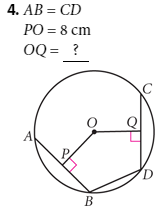# Lesson 9.2 Chord Properties 2

Topic:
Circle

## Lesson 9.2: Chord Properties 2

1. Drag point C and observe the relationship between the chord and the line perpendicular to it from the center of the circle. At what special point does the perpendicular line intersect the chord? Write a conjecture (Perpendicular to a Chord Conjecture). 2. Measure the distance from point A to BC and the distance from point A to DE. Drag parts of your sketch and observe these distances for different pairs of congruent chords. Write a conjecture about congruent chords in a circle and their distances from the circle’s center (Chord Distance to Center Conjecture).

Perpendicular to a Chord Conjecture: The perpendicular from the center of a circle to a chord is the ________ of the chord.

Select all that apply
• A
• B
• C

Chord Distance to Center Conjecture: Two congruent chords in a circle are ____________ from the center of the circle.

Select all that apply
• A
• B
• CSelect all that apply
• A
• B
• C